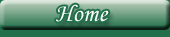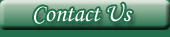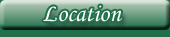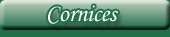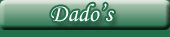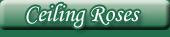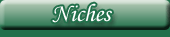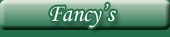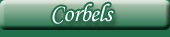# Plaster Niches

Size Key;

H = Height.

W = Width.

D = Depth.

All measurements are in inches. Images are not to scale.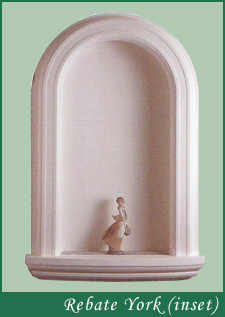H = 45.5". W = 30". D = 5"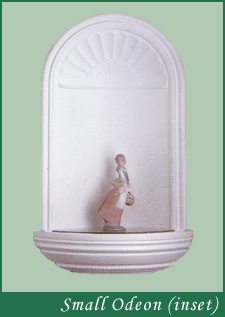H = 33". W = 18.25". D = 4"H = 38". W = 25.5". D = 6"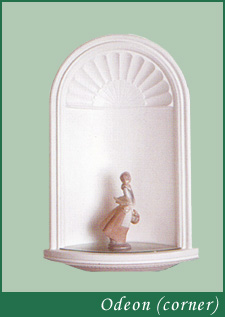H = 30.5". W = 18.25".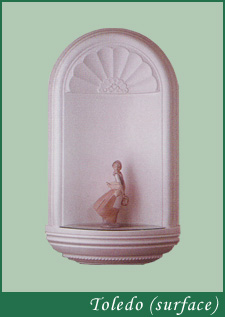H = 35.5". W = 20".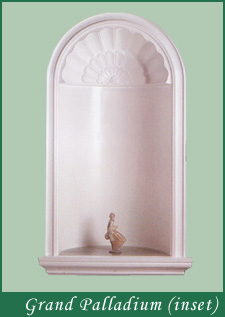H = 62". W = 36". D = 15"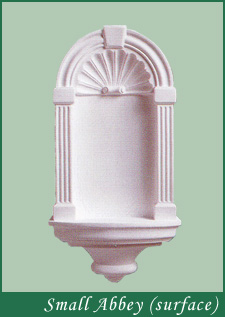H = 21.75". W = 11".

NEW THIS YEAR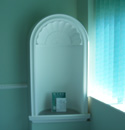Name Here  H = XX". W = XX". D = XX"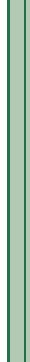Website by MWDUK.com